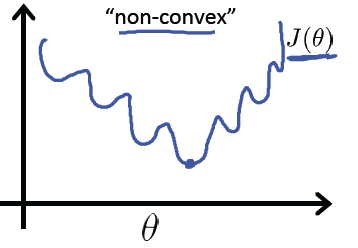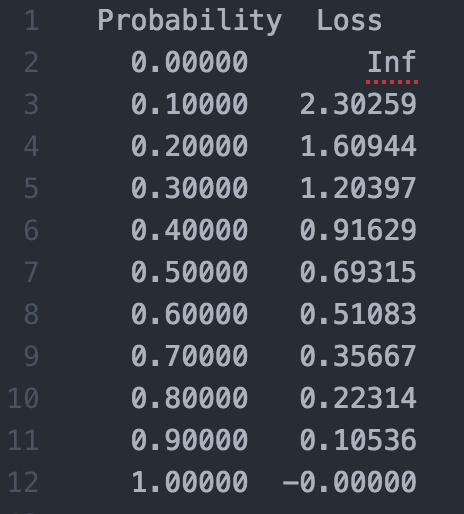## 提纲

1. 分类模型 与 Loss 函数的定义
2. 为什么不能用 Classification Error
3. Cross Entropy 的效果对比
4. 为什么不用 Mean Squared Error
5. 定量理解 Cross Entropy
6. 总结
7. 参考资料

## 分类模型 与 Loss 函数的定义

• 分类问题：目标变量是离散的。
• 回归问题：目标变量是连续的数值。

## 为什么不能用 classification error

$$\text{classification error} = \frac{\text{count of error items}}{\text{count of all items}}$$

computed 一栏是预测结果，targets 是预期结果。 二者的数字，都可以理解为概率。 correct 一栏表示预测是否正确。

computed targets correct?
0.3 0.3 0.4 0 0 1 (democrat) yes
0.3 0.4 0.3 0 1 0 (republican) yes
0.1 0.2 0.7 1 0 0 (other) no

item 1 和 2 以非常微弱的优势判断正确，item 3 则彻底错误。

$$\text{classification error} = 1/3 = 0.33$$

computed targets correct?
0.1 0.2 0.7 0 0 1 (democrat) yes
0.1 0.7 0.2 0 1 0 (republican) yes
0.3 0.4 0.3 1 0 0 (other) no

item 1 和 2 的判断非常精准，item 3 判错，但比较轻。

$$\text{classification error} = 1/3 = 0.33$$

2 个模型的 classification error 相等，但模型 2 要明显优于模型 1.

classification error 很难精确描述模型与理想模型之间的距离。

## Cross-Entropy 的效果对比

TensoFlow 官网的 MNIST For ML Beginners 中 cross entropy 的计算公式是：

$$H_{y'}(y) := -\sum_{i}y'_i \log(y_i)$$

$$-( (ln(0.3)*0) + (ln(0.3)*0) + (ln(0.4)*1) ) = -ln(0.4)$$

$$-(ln(0.4) + ln(0.4) + ln(0.1)) / 3 = 1.38$$

$$(ln(0.7) + ln(0.7) + ln(0.3)) / 3 = 0.64$$

ACE 结果准确的体现了模型 2 优于模型 1。

cross-entropy 更清晰的描述了模型与理想模型的距离。

## 为什么不用 Mean Squared Error (平方和)

$$(0.3 - 0)^2 + (0.3 - 0)^2 + (0.4 - 1)^2 = 0.09 + 0.09 + 0.36 = 0.54$$

$$(0.54 + 0.54 + 1.34) / 3 = 0.81$$

$$(0.14 + 0.14 + 0.74) / 3 = 0.34$$

MSE 看起来也是蛮不错的。为何不用?

$$softmax(x)_i = \frac{\exp(x_i)}{\sum_j \exp(x_j)}$$cross entropy 计算 loss，则依旧是一个凸优化问题，## 总结

training 过程中，分类问题用 cross entropy，回归问题用 mean squared error。

training 之后，validation / testing 时，使用 classification error，更直观，而且是我们最关注的指标。